GFG App
Open AppBrowser
Continue

# Methods of calculation of Standard Deviation in frequency distribution series

A scientific measure of dispersion that is widely used in statistical analysis of a given set of data is known as Standard Deviation. Another name for standard deviation is Root Mean Square Deviation. It is denoted by a Greek Symbol σ (sigma). Under this method, the deviation of values is taken from the arithmetic mean of the given set of data. Standard Deviation is considered to be the best way of determining the dispersion of a data set. It is because standard deviation takes into account every value of a data set along with its algebraic signs. It can be calculated in three different series; viz., Individual, Discrete, and Frequency Distribution Series.

According to Spiegel, “The Standard Deviation is the square root of the arithmetic mean of the squares of all deviations. Deviations being measured from arithmetic mean of the items.”

## Methods of Calculating Standard Deviation in Frequency Distribution Series

1. Direct Method
2. Short-Cut Method
3. Step-Deviation Method

### 1. Direct Method

In this method, the standard deviation of a series of data is determined directly through deviations of the arithmetic mean from each value. The steps of calculating the standard deviation of discrete series with the direct method are as follows:

Step 1: First of all, the arithmetic mean of the given series or data set is determined. It is denoted byStep 2: Now, the deviations of every mid-value of the class intervals or size are taken from the arithmetic mean, i.e.,Step 3: In the next step, the deviations determined are squared and then multiplied by their respective frequencies, resulting in fx2

Step 4: The last step is to use the formula of the direct method to calculate the standard deviation.OR#### Example:

Calculate the standard deviation of the following series by Direct Method.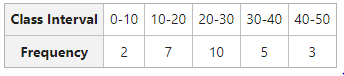#### Solution: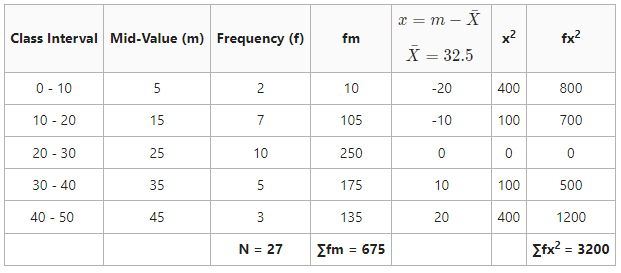= 10.89

Standard Deviation = 10.89

### 2. Short-Cut Method

In this method, the standard deviation of a series of data is determined by obtaining deviations of the mid-values of the class intervals of a data set. The deviation of the mid-values is taken from the arithmetic mean of the data set. The steps of calculating the standard deviation of frequency distribution series with the short-cut method are as follows:

Step 1: First of all, a value is assumed from the mid-values of the given data set and then the deviations of the assumed value are taken from the mid-values. The deviation is denoted by d, where d = m – A.

Step 2: Now, the frequencies of the data set are multiplied by their respective deviations and are denoted by fd.

Step 3: In the next step, the fd determined in the previous step is multiplied by the deviations (d).

Step 4: The last step is to calculate the standard deviation of the frequency distribution series using the formula.#### Example:

Calculate the standard deviation of the following series by the Short-Cut Method.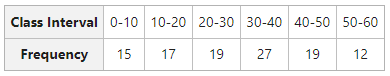#### Solution: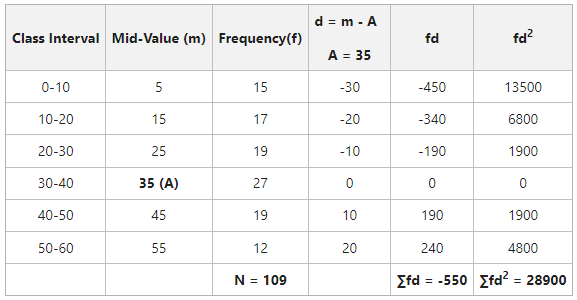= 15.48

Standard Deviation = 15.48

### 3. Step-Deviation Method

In this method, the standard deviation of a series of data is determined by taking a common factor of the class intervals into consideration. It is the most popular method of determining standard deviation. The steps to calculate the standard deviation of a frequency distribution series by the Step-Deviation Method are as follows:

Step 1: First of all, a value is assumed from the mid-values of the given data set, and then the deviations of the assumed value are taken from the mid-values. The deviation is denoted by d, where d = m – A.

Step 2: The next step is to divide the deviations by their common factor, denoted by d’, where.

Step 3: Now, d’ is multiplied by their respective frequencies to get fd’.

Step 4: In the next step, fd’ is multiplied by d’ to get fd’2.

Step 5: The last step is to calculate the standard deviation of the frequency distribution series using the formula.#### Example:

Calculate the standard deviation of the following series by Step-Deviation Method.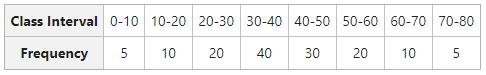Solution: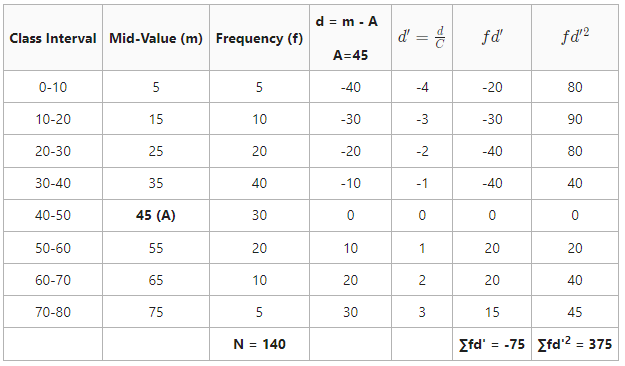= 15.46

Standard Deviation = 15.46

My Personal Notes arrow_drop_up